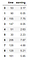# Machine learning model for predicting medium writer earnings

On October 22, 2019, Medium unveiled a new model for calculating writer’s earnings. According to this new model, earnings will be calculated based on the reading time of Medium members. You may find out more about the new model from this article: Improving how we calculate writer earnings.

The new model took effect as of October 28, 2019. In a previous article (Medium Partner Program’s New Model for Calculating Writer’s Earnings — Linear Regression Analysis), I had written about a model for writer earnings under the new Partner Program model. However, this article is based on 5 days of data (October 28, 2019 to November 2, 2019) only.

Since the new model is operational now for 1 month now, I decided to revisit the problem of estimating writer earnings under the new Partner Program, this time using 1 month of data (my November earnings data).

In this article, we build a simple model using the earnings_data.csv dataset for predicting writer’s daily earnings based on member reading time. This article is organized in 6 sections as follows: (1) importation of necessary libraries; (2) importation of dataset; (3) building of regression model; (4) visualization of fitted regression line; (5) model training, testing, and evaluation; and (6) summary of model findings and conclusions.

# 1. Import necessary libraries

`import numpy as npimport pandas as pdimport matplotlib.pyplot as pltfrom sklearn.metrics import r2_scorefrom sklearn.model_selection import train_test_split`

# 2. Read dataset and display columns

`df=pd.read_csv("earnings_data.csv")df.head(n=10)`Dataset has 2 features: time (daily member’s reading time in minutes) and earning (daily earning per story).

# 3. Build basic regression model

`X = df['time'].valuesy = df['earning'].valuesslope = np.polyfit(X,y,1)intercept = np.polyfit(X,y,1)y_pred = intercept + slope*XR2_score = r2_score(y, y_pred)`

# 4. Visualization of fitted regression line

`plt.figure(figsize=(8,6))plt.scatter(X,y,label='data', c='steelblue', edgecolor='white', s=150)plt.plot(X, y_pred,color='black', lw=2,label='fit')plt.title('\$ R^2 = 0.939 \$',size=14)plt.xlabel('daily members reading time per story (min)',size=14)plt.ylabel('daily earning per story (\$)',size=14)plt.legend()plt.show()`

# 5. Model training, testing, and evaluation

Here, we perform model training, testing, and evaluation (using cross-validation) to make sure model is robust and stable. For cross-validation analysis, we generated 10 random samples of our training and testing sets.

`slope = []intercept =  []train_score = []test_score = []for i in range(10):    X_train, X_test, y_train, y_test = train_test_split( X, y, test_size=0.4, random_state=i)    a = np.polyfit(X_train,y_train,1)    b = np.polyfit(X_train,y_train,1)    y_train_pred = a*X_train + b    y_test_pred = a*X_test + b    train_score = np.append(train_score,                             r2_score(y_train,y_train_pred))    test_score = np.append(test_score, r2_score(y_test,y_test_pred))    slope = np.append(slope, np.polyfit(X_train, y_train,1))    intercept = np.append(intercept,                           np.polyfit(X_train, y_train,1))`

Observations: Looking at the outputs above, we see that the model is pretty robust and stable. We also calculated the mean slope of the regression line to be 0.038 and the mean intercept to be 0.818.

# 6. Summary of model findings and conclusions

Based on our machine learning model, we found a relationship between daily earnings per story(\$) and daily members reading time (in minutes):

## earnings = 0.038 x time + 0.818

Note: This relationship would only hold for member reading time in the range from 1 minute to 900 minutes, the range use for training the model. Because the slope is a random variable described by slope = 0.38 +/- 0.001, we expect that the model when used, will produce small variations in the predicted data. You may expect this model to produce variations in the predicted earnings of about +/- \$1.

In summary, we’ve shown how a simple linear regression model can be used to predict writer’s earnings using the partner program’s newest model. We hope to extend this calculation in the future as more and more earnings data become available from the new medium partner program model. In the meantime, this model can serve as a reliable model for estimating daily earnings from members reading time.

Written by

## Towards AI

#### Towards AI, is the world’s fastest-growing AI community for learning, programming, building and implementing AI.

Welcome to a place where words matter. On Medium, smart voices and original ideas take center stage - with no ads in sight. Watch
Follow all the topics you care about, and we’ll deliver the best stories for you to your homepage and inbox. Explore
Get unlimited access to the best stories on Medium — and support writers while you’re at it. Just \$5/month. Upgrade